Home » Physics » Acceleration formula Explained with Examples

# Acceleration formula Explained with Examples

## Acceleration Formula

Acceleration is the rate of change of velocity with time. It is a measure of the change in the velocity of an object per unit time and mathematically acceleration formula  is given as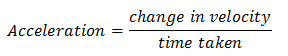For describing average acceleration, we first consider the motion of an object along a straight line.
Suppose at time t1 object is at point P moving with velocity v1 and at time t2 it is at point Q and has velocity v2. Now average acceleration of an object in moving from P to Q is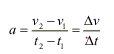which is the change in velocity of the object with the passage of time.

If the velocity of an object changes from an initial value u to the final value v in time t, the acceleration a is given by,
a=(v-u)/t
and this kind of motion is called accelerated motion.

1) Since the velocity of a moving object has both magnitude and direction likewise acceleration depending on velocity has both magnitude and direction and hence acceleration is a vector quantity.

2) SI unit of acceleration is ms-2

3) A body has uniform acceleration if it travels in a straight line and its velocity increases by an equal amount in equal intervals of time for example freely falling bodies, the motion of the ball rolling down the inclined plane etc.

4) A body has non-uniform acceleration if its velocity increases or decreases by the unequal amount in equal intervals of time.

5) If acceleration is in the direction of the velocity then it is positive acceleration and if it is in the direction opposite to the direction of velocity then it is negative and the negative acceleration is termed retardation or deceleration.

Example -1
A motorbike moves in a straight road. The velocity at point A is 36 km/hr, It presses the accelerator paddle and reaches point B in 10 sec. The velocity at point B is 54Km/hr
Find the average acceleration of the car from point A to point B?
Solution
Average acceleration is given byInitial velocity= 36 km/hr=10 m/s
Final Velocity = 54 km/hr=15 m/s
Average acceleration= (15-10)/10= .5 m/s2
Example -2
True and False Statement
a) Acceleration is a scalar quantity?
b) if the velocity of the object is zero, then acceleration will always be zero
c) If a car is moving fast, does it always have acceleration?
Solution
a) False. Acceleration is vector quantity
b) False.
c) False. Fast does not mean acceleration always. It may be moving with high velocity but velocity may not be changing

## Motion with constant acceleration

Motion with constant acceleration or uniformly accelerated motion is that in which velocity changes at the same rate throughout the motion.
Here are the equations for uniformly accelerated motion in a straight line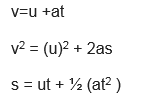Where
v=Final Velocity
u=Initial Velocity
a= Constant acceleration
t= time interval
s= displacement

Example -3
A train starts from rest and accelerates uniformly at the rate of 5 m/s2  for 10 sec.
a) Calculate the velocity of the train in 10 sec.
b) Distance traveled by train in 10 sec
Solution
Here it is the example of uniformly accelerated motion
a=5 m/s2
u=0
t=10 sec
v=?
s=?
From
v=u +at
v= 0 + 5×10
=50 m/sec
From
s = ut + ½ (at2 )
s= ½ (5×102 )=250 m

## Instantaneous acceleration formula

The acceleration of the moving body at any instant of time is defined as its instantaneous acceleration.
Instantaneous acceleration can be defined in the same way as instantaneous velocity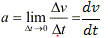The instantaneous acceleration at any instant is the slope of v-t graph at that instant.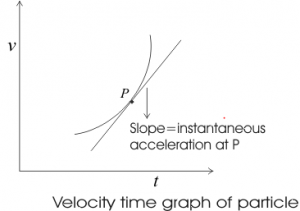Above figure shows instantaneous acceleration at point P is equal to the slope of the tangent at this point P.

Example
A train is moving on the rail track. The distance traveled by it is defined by the following equation:
x = t3 + t + 4
Find the instantaneous acceleration at time t = 1 sec?
SolutionNow
x = t3 + t + 4
We know that v= dx/dt
So
v= 3t2+1
Acceleration would be given by
a =6t
So, instantaneous acceleration at time t = 1 sec
= 6 m/s2

### How to solve problems involving time-dependent acceleration

Here I tried to sum up the steps to solve problems involving time-dependent acceleration. We can easily calculate the position of the particle/object

1. Consider that acceleration a is some function of time such that
$a=f(t)$
this equation can also be written in terms of velocity i.e.
$a=\frac{dv}{dt}=f(t)$                                        …..1
2. Now we integrate equation 1 as a function of time
$dv=f(t)dt$
$v=\int dv=\int f(t)dt +C$               ……2
here C is some constant of integration and can be calculated from the initial conditions.
3. Now we know that $v=\frac{dx}{dt}$ , using this in the solution of equation 2 we can easily find the position of the particle.
Practice Questions on Acceleration Formula with Distance
Question 1
On a highway with speed limit 80km/h, a car was stopped by applying brakes with a retardation of 5m/s2 in 5 seconds. What is the initial velocity of the car? Was it over-speeding?
Question 2
A cycle traveling with a velocity of 20km/h accelerates at the rate of 0.10m/s2. After what time will it achieve a velocity of 38km/h?
Question 3
A truck moves with a velocity of 36km/h. How much distance will it cover in 1 minute if it moves with a uniform acceleration of 1m/s2?
Question 4
A car starts and attains a velocity of 60m/s in the 30s. If the acceleration is uniform, what is the distance traveled by car to achieve this velocity?
Question 5
A car travelling with a velocity of 10m/s stops on the application of brakes. It produces a uniform retardation of 1.25m/s2. Calculate the distance traveled by car before it comes to rest. Also, find the time taken by the car to stop.
Question 6
How much distance will a vehicle move with a uniform acceleration of 4m/s2 cover in 5 seconds if the initial velocity of the vehicle is 5m/s?Question 7
A bullet leaves a rifle with a muzzle velocity of 521m/s. While accelerating through the barrel of the rifle, the bullet moves a distance of 0.840 m. Determine the acceleration of the bullet (assume a uniform acceleration).Question 8
The observation deck of the tall skyscraper 370 m above the street. Determine the time required for a penny to free fall from the deck to the street below.Question 9
Honda Activa accelerates uniformly from rest to a speed of 7.10 m/s over a distance of 35.4 m. Determine the acceleration of the bike.

Question 10

If a car has a constant acceleration of 4 m/s2, starting from rest, how fast is it traveling after 5 seconds?

Question 11
What is the acceleration of Honda with a constant velocity of 50 km/h for 20 seconds? Does the car have a constant acceleration?

Question 12
A boy on a bicycle increases his velocity from 5 m/s to 20 m/s in 10 seconds.
a) What is the acceleration of the bicycle?
b) What distance was covered by the bicycle during the 10 seconds?

## Acceleration Direction

Acceleration is a vector quantity so it has both magnitude and direction. Direction of acceleration is in the direction of velocity if it is increasing and opposite to the direction of velocity if the velocity is decreasing

Now we use + and – to specify direction in physics. + stands for positive direction and – negative stands for the opposite of positive dimension

if the velocity is in positive direction and it is increasing , acceleration will be positive direction and will be positive

Example
u= 2 m/s ( + as positive direction)
v=3m/s  ,t=1 sec
a= (3-2)/1= 1m/s2
o acceleration is positive

if the velocity is in positive direction and it is decreasing, acceleration will be negative direction and will be negative

Example
u= 2 m/s ( + as positive direction)
v=1m/s  ,t=1 sec
a= (1-2)/1= -1m/s2
So acceleration is negative

if the velocity is in negative direction and it is increasing , acceleration will be negative direction and will be negative

Example
u= -2 m/s ( – as negative direction)
v=-3m/s  ,t=1 sec
a= (-3+2)/1= -1m/s2
So acceleration is negative

if the velocity is in negative direction and it is decreasing , acceleration will be positive direction and will be positive

Example
u= -2 m/s ( – as negative direction)
v=-1m/s  ,t=1 sec
a= (-1+2)/1= 1m/s2
So acceleration is positive.

## Acceleration in Curvilinear Motion

So far we have seen acceleration examples in One-dimensional motion. Now we will study the acceleration in Curvilinear motion. Curvilinear motion happens when object or particles move along a specific curve path. It can be two dimensional or Three dimension.
The basic principle of acceleration remains same but we have to deal with 2 or 3 direction in curvilinear motion.
Please look at the below links to get a good knowledge of Acceleration in Two or Three-dimensional motion. It provides acceleration formula for those motion also. This also contains an example to solve such a problem

## Centripetal Acceleration Formula

when a particle moves along a circular path it must have components of acceleration perpendicular to the path when its speed is constant. Such type of circular motion is called Uniform circular motion

The magnitude of the instantaneous normal acceleration is equal to the square of the speed divided by the radius. Its direction is perpendicular to velocity vector and acts along an inward direction along the radius of the circular path towards the center of the circle.

Because of this, it is called central or centripetal acceleration. The term centripetal means seeking the center.

Centripetal Acceleration Formula = v2/R

Where v is the speed of the object and R is the radius of the circular path

Circular Motion Physics: Detailed explanation

Acceleration in a curvilinear motion

Circular Motion Physics: Detailed explanation

Motion in straight line practice problems for class 11

Hope you like the detailed explanation with examples for acceleration formula. Download of whole article is also given below

Acceleration-formula
acceleration-formula.pdf
318.3 KiB